×#### Thank you for registering.

One of our academic counsellors will contact you within 1 working day.

Click to Chat

1800-1023-196

+91-120-4616500

CART 0

• 0

MY CART (5)

Use Coupon: CART20 and get 20% off on all online Study Material

ITEM
DETAILS
MRP
DISCOUNT
FINAL PRICE
Total Price: Rs.

There are no items in this cart.
Continue Shopping• Complete JEE Main/Advanced Course and Test Series
• OFFERED PRICE: Rs. 15,900
• View Details

```Chapter 6: Graphs of Trigonometric Functions – Exercise 6.2

Graphs of Trigonometric Functions – Exercise 6.2 – Q.1

We have,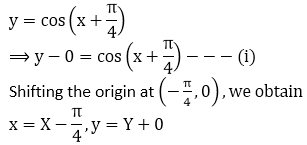Substituting these values in (i), we get

Y = cost X.

Thus we draw the graph of Y = cos X and shift it by π/4 to the left to get the required graph.We have,Substituting these values in (i), we get

Y = cos X.

Thus we draw the graph of Y = cos X and shift it by π/4 to the left to get the required graph.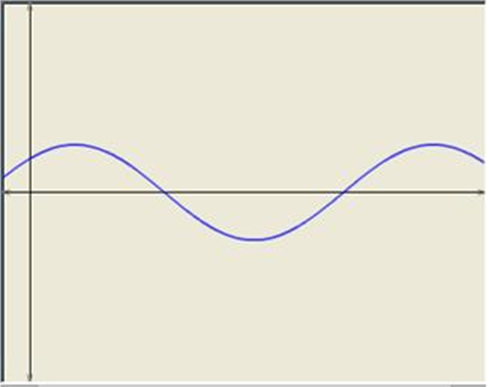We have,Y = 3 cos 2X.

Thus we draw the graph of Y = 3 cos 2 X and shift it by 1/2 to the left to get the required graph.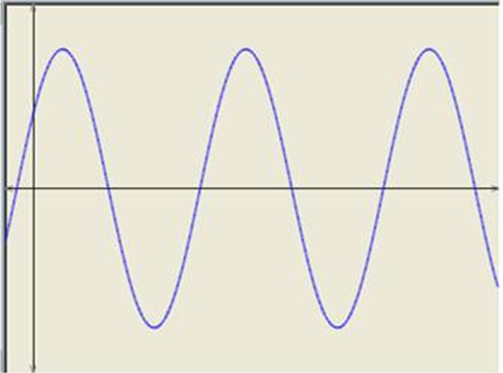We have,Substituting these values in (i), we get

Y = 2 cos X.

Thus we draw the graph of Y = 2 cos X and shift it by π/2 to the left to get the required graph.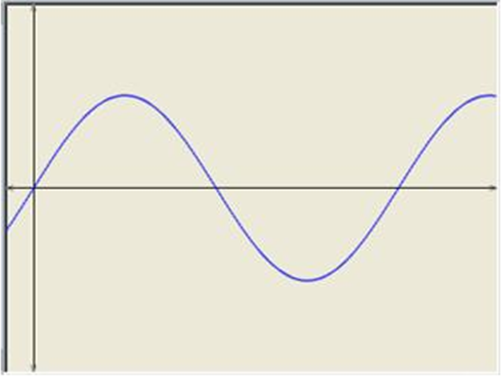Graphs of Trigonometric Functions – Exercise 6.2 – Q.2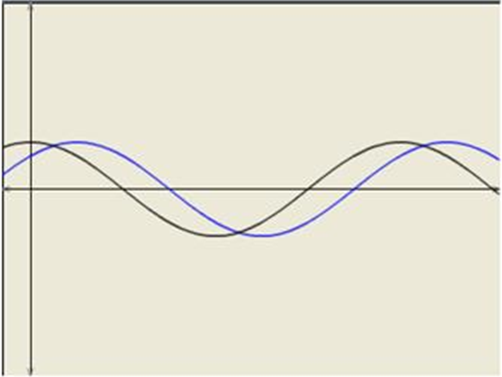We have,Substituting these values in (i), we get

Y =  cos 2X.

Thus we draw the graph of Y = 2 cos X and shift it by π/4 to the left to get the required graph.To obtain the graph of y = cos x/2 we first draw the graph of y = cos x in the interval [0, 2π] and then divide the x-coordinates of the points where it crosses x-axis by 1/2.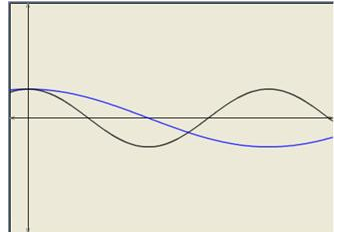```### Course Features

• 728 Video Lectures
• Revision Notes
• Previous Year Papers
• Mind Map
• Study Planner
• NCERT Solutions
• Discussion Forum
• Test paper with Video Solution